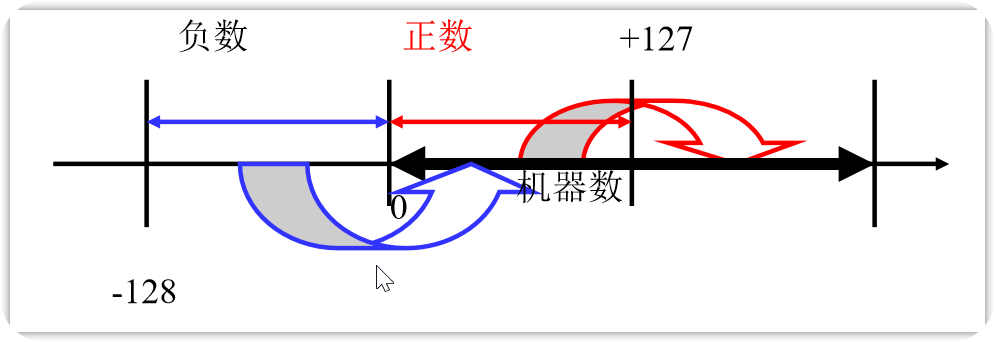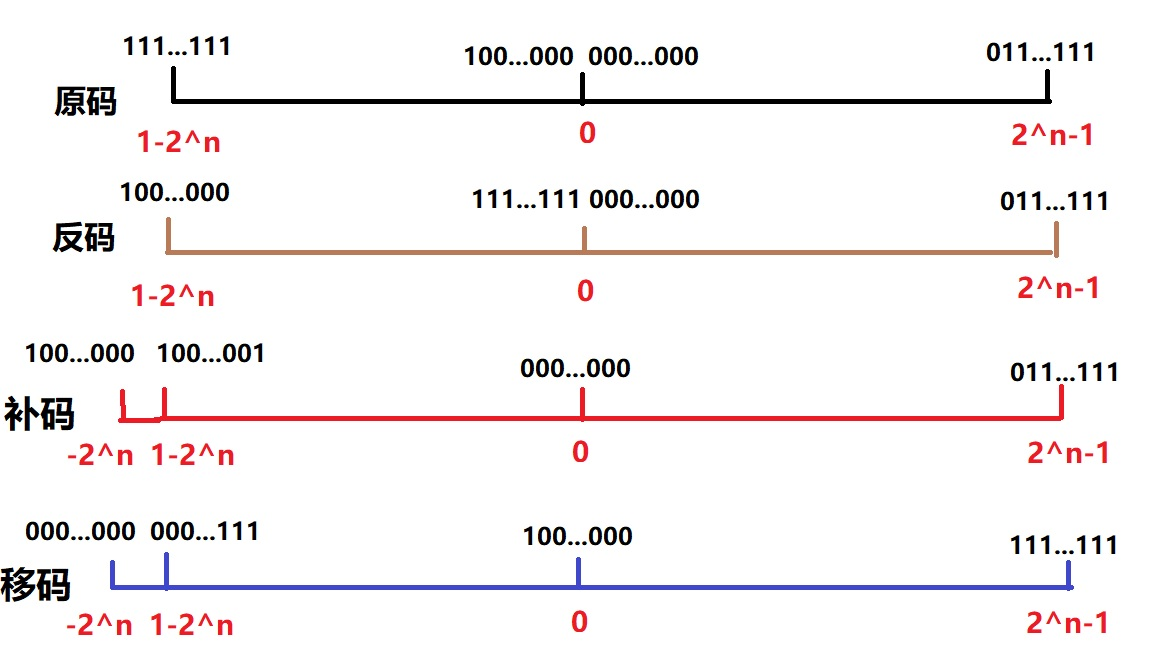# 数的机器码表示——彻底弄清什么是原码、反码、补码、移码

2019/04/10 10:10

## 数的机器码表示

@[toc] 为了妥善的处理数据运算过程中符号位的问题，于是就产生了把符号位和数值位一起编码起来表示相应的数的各种表示方法。例如我们熟悉的原码、反码、补码、移码等。通常将未经编码的数称为真值，编码后的数称为机器数或者机器码

• 真值的形式正、负号加某进制数绝对值的形式，即数的实际值。如+3-5

• 机器数的形式：真值按某种编码方式进行编码后的数值，即真值在机器中的表示，称为机器数，一般可以分为 无符号数和有符号数两种如：X=01011Y=11011

### 原码

#### 定点整数

$x=-7$,化为二进制表示为$x=-0111$;$x_{[原]}=2^3-(-0111)=1000+0111=1111$。

• 对于正数$x=+x_{n-1}...x_1x_0$,它的原码是它自己本身，常常在最高位前面补0，代表它是一个正数。

• $x_{[原]}=0x_{n-1}...x_1x_0$
• 对于0，根据原码的定义则有两种表示形式：

• $+0=+0_{n-1}...0_10_0$
• 此时正0的原码为$+0_{[原]}=00_{n-1}...0_10_0$
• $-0=-0_{n-1}...0_10_0$
• 此时负0的原码为$-0_{[原]}=10_{n-1}...0_10_0$
• 对于负数$x=-x_{n-1}...x_1x_0$,它的原码是在最高位前面补1，代表它是一个负数。

• $x_{[原]}=-x_{n-1}...x_1x_0$

#### 定点小数

$x=-0.875$二进制表示为$x=-0.111$;$x_{[原]}=1.000-(-0.111)=1.000+0.111=1.111$。 我们可以总结出来：

• 对于正数$x=+0.x_{n-1}...x_1x_0$,它的原码是它自己本身，常常在最高位前面补0，代表它是一个正数。(注意，前面的0.实际上是不存储的，也就是实际最高位是$x_{n-1}$)

• $x_{[原]}=0.x_{n-1}...x_1x_0$
• 对于0，根据原码的定义则有两种表示形式：

• $+0=+0.0_{n-1}...0_10_0$
• 此时正0的原码为$+0_{[原]}=0.0_{n-1}...0_10_0$
• $-0=-0.0_{n-1}...0_10_0$
• 此时负0的原码为$-0_{[原]}=1.0_{n-1}...0_10_0$
• 对于负数$x=-0.x_{n-1}...x_1x_0$,它的原码是在最高位前面补1，代表它是一个负数。(注意，前面的0.实际上是不存储的，也就是实际最高位是$x_{n-1}$)

• $x_{[原]}=-1.x_{n-1}...x_1x_0$

#### 原码的计算

​ 原码虽然表示极为简单，但是最大的问题就是加法运算十分复杂

### 反码

$1-1=0$

$0001-0001=0000$

$1+(-1)=0$

$0001+1001=1010=-2$

#### 定点整数

• +0
• 0000-->0000
• -0
• 1000-->1111

#### 反码的运算

$1+(-1)=0$

0001+1110=1111=-0

10000001+01111111=100000000

• 进了一位，末位加一
• 10000000+01111111+1
• 最后结果为00000001

### 补码

#### 补码的由来

<img src="https://cdn.jsdelivr.net/gh/c1ata/imgbed2020/img/image-20200318143453928.png" alt="image-20200318143453928" style="zoom: 33%;" /><img src="https://cdn.jsdelivr.net/gh/c1ata/imgbed2020/img/image-20200318143525840.png" alt="image-20200318143525840" style="zoom:33%;" />

$5-2=5+10（MOD 12） \5+（-2）=5+10（MOD 12）\ -2=10（MOD 12）$ 在上面的式子中，在模为12的情况下，-2的补码就是10。一个负 数用其补码代替，同样可以得到正确的运算结果。

#### 定点整数

$x=-7$,化为二进制表示为$x=-0111$;$x_{[补]}=2^4+(-0111)=10000-0111=1001$。

• 对于正数$x=+x_{n-1}...x_1x_0$,它的补码是它自己本身，常常在最高位前面补0，代表它是一个正数。

• $x_{[补]}=0_nx_{n-1}...x_1x_0$

• 对于0，根据补码的定义：

• $+0=+0_{n-1}...0_10_0$

• 此时正0的补码为$+0_{[补]}=0_n0_{n-1}...0_10_0$
• $-0=-0_{n-1}...0_10_0$

• 此时负0的补码为$-0_{[补]}=（10_n0_{n-1}...0_10_0-0_{n-1}...0_20_10_1）mod(10_n0_{n-1}...0_10_0)=0_n0_{n-1}...0_10_0$

由此可见，零的补码是唯一的，没有+0和-0之分。

• 对于负数$x=-x_{n-1}...x_1x_0$

​ 常常可以通过把数值位按位取反，然后末位加一来计算负数的补码

• 如求$-127$的补码
• $[-0111 1111]_{[补]}=10000 0000-01111111$
• $10000000=11111111+1$
• $[-0111 1111]_{[补]}=11111111-01111111+1$，这一步刚刚说明了上面的计算方法的原理。

$-128$的补码的求法。

• -128=-1000 0000
• $[-10000000]_{[补]}=11111111-10000000+1=10000000$

其实还有一个更为渐变的补码的求法：**从右到左遇到第一个 1 的前面各位取反。**也就是从右向左，遇到1之前，还保持原样。遇到1之后，各位取反。以-126为例：$-126=-01111110$

[-01111110]的补码位10000010，10是不变的。而黑体部分则是取反的。

00000000~011111111 ——0~127

#### 定点小数

$x=-0.875$二进制表示为$x=-0.111$;$x_{[补]}=10.000-(0.111)=1.001$。

• 对于正数$x=+0.x_{n-1}...x_1x_0$,它的补码是它自己本身，常常在最高位前面补0，代表它是一个正数。(注意，前面的0.实际上是不存储的，也就是实际最高位是$x_{n-1}$)

• $x_{[原]}=0.x_{n-1}...x_1x_0$

• 对于0，根据补码的定义：

• $+0=+0.0_{n-1}...0_10_0$

• 此时正0的补码为$+0_{[补]}=0.0_{n-1}...0_10_0$
• $-0=-0.0_{n-1}...0_10_0$

• 此时负0的补码为$-0_{[补]}=（10.0_{n-1}...0_10_0-0.0_{n-1}...0_10_0）mod(10.0_{n-1}...0_10_0)=0.0_{n-1}...0_10_0$

也就是说**，0的补码是唯一的**。

• 对于负数

按位取反，末位加一，和定点整数一样。虽然我们看起来有一个小数点，但是实际上小数点是不存储的。

1000~1111--> [-1,-0.125]

#### 补码的运算

$X_{真值}=-2^n\times x_n+\sum_{0}^{n-1}2^ix_n$

$X_{真值}=\sum_{0}^{n-1}2^ix_n$

$X_{真值}=-2^n+\sum_{0}^{n-1}2^ix_n$

• 以-127为例，-127=-01111111
• 它的补码为10000001
• 从补码求真值的过程:-10000000+1=-01111111-1+1=-01111111

$126-127=0$

• 原码

• $01111110-01111111$

• 我们需要比较数值位的绝对值大小，来决定符号位，1

• 1111111-1111110=0000001

• 10000001

假设把减法看成加法

• 01111110+（-01111111）=01111110+11111111=100000001
• 1会溢出，那么得到的结果就是00000001，按原码表示的化，真值为1，显然是错误的。
• 补码

• 01111110+10000001=11111111
• 转换为真值后为-1。正确的
• 原理是这样的
• 126-127=-1
• 126+(-127)=-1
• 126+129mod256=-1
• 这里-127等效于129mod256

• 01111111
• 10000001

### 移码

$[e]_移=2^k+e$

-8 1000 0000
-7 1001 0001
-6 1002 0002
…… ...... ......
0 0000 1000
+1 0001 1001
…… ...... .......
+7 0111 1111

#### 移码的表示#### 移码的特点

• 在移码中，最高位为0表示负数，最高位为1表示正数，这与原 码、补码、反码的符号位取值正好相反
• 移码为全0时所对应的真值最小，为全1时所对应的真值最大！ 因此，移码的大小直观地反映了真值的大小，这将有助于两个 浮点数进行阶码大小比较
• 真值0在移码中的表示形式是唯一的，即：[+0]移= 移= 100…00
• 移码把真值映射到一个正数域，所以可将移码视为无符号数， 直接按无符号数规则比较大小。
• 同一数值的移码和补码除最高位相反外，其他各位相同。

### 原码、反码、补码、移码0
0 收藏

### 作者的其它热门文章0 评论
0 收藏
0